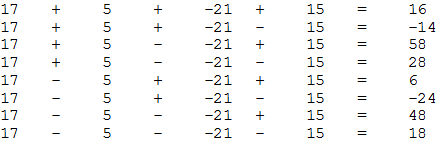You are given a sequence of numbers and a positive integer K. You can place a plus(+) or a minus(-) between integers in the sequence. A sequence is K-divisible if + and - can be placed in at least one way that the expression is divisible by K. N is the number of integers in the sequence. For N numbers in the sequence, there will be 2^(N-1) different arrangements of + and -.

Eg 17, 5, -21, 15 and K = 7 N = 4So the sequence is 7-divisible (-14 or 28) but is not 10-divisible.

Input
First line contains the number of test cases T. Each test case has two lines. First line contains two integers N (number of integers in the sequence) and K.

Output
Output a single line for each test case as `Divisible` or `Not divisible`

Constraints
1 <= T <= 20
1 <= N <= 30
2 <= K <= 100

Sample Input``` 2 4 7 17 5 -21 15 4 10 17 5 -21 15```

Sample Output``` Divisible Not divisible ```

Languages: Brain,C,C++,Java,Pascal,Perl,PHP,Python,Ruby,Text

Time Limit: 2 Second(s)
Score: 0 Point(s)
Input File Limit: 50000 Bytes

Mode Judge

RankNameScore
1xyz0
2Ams0
3TIP0
4team420
5xyzz0
6asdasdasd0
7abcd0
8khankhan0
9Gabriel0
10gigel0